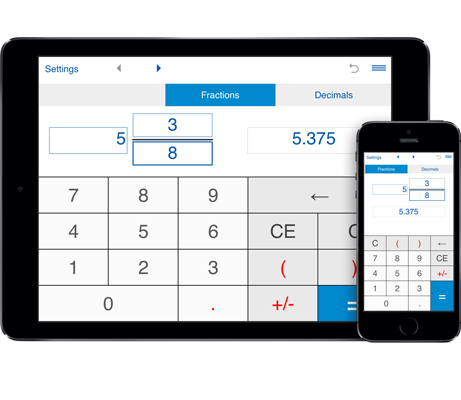# Decimal to fraction converter

Decimal to fraction converter will easily convert numbers to fractions and vice versa.
You can use this calculator for both terminating decimals and repeating decimals.
For repeating decimals enclose the repeating digits in parenthesis. Eg. type 0.24(3) for 0.24333... If the number is 5.123123..., type 5.(123).

Decimal to Fraction Converter Settings

Number of decimal places

Round fraction to the nearest

## Convert decimal numbers to fractions

To convert a decimal number to a fraction, select "decimal to fraction", enter a decimal number using the decimal point "." as separator (eg. 1.23) and press "=" to calculate the equivalent fraction.
Calculator for converting decimal numbers to fractions and fractions to decimals
This decimal to fraction calculator will quickly convert decimals to fractions. You can use it for both regular decimals and recurring decimals.

The decimal to fraction converter shows the results as a fraction or mixed number in simplest form.

### Decimal to Fraction Conversion Calculator

To convert a decimal to a fraction using the conversion calculator:

1. Select the "decimal to fraction" option.

2. Enter a decimal number you want to convert to a fraction and press "=".

The calculator will convert a decimal to an improper fraction, reduce that fraction if it can be reduced, and show the results as a proper fraction or mixed number in simplest form.

Decimals input:

Enter decimals in the following formats:

When a number has no repeating decimal portion (terminating decimals or non-repeating decimals), enter the number with the whole part separated from the decimal part by a decimal point (ex. 12.34).

When a number has infinitely repeating decimals (or recurring decimals) you should enclose the repeating digits in brackets. Ex. if you have 0.24333... as decimal, type 0.24(3). If the number is 0.123123, type 0.(123).